## Logic 1

Recall that in Engr 121 we used arithmetic operations instead of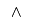,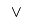and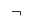1. What was the symbol for each?should be natural, the others a little forced
2. Draw a truth table for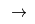and determine which arithmetic operation is appropriate An inequality

## Logic 2

On first glance, the statements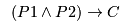and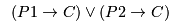appear to say different things.
1. Compute a truth table for both. What is the result?
P1 P2 C1 1 1 1 1
1 1 0 0 0
1 0 1 1 1
1 0 0 1 1
0 1 1 1 1
0 1 0 1 1
0 0 1 1 1
| 0 | 0 | 0 | 1 | 1 |
2. Similarly, for each statement, find all situations where it is false. What do you see here?
1. The only timeis false is when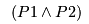is true and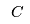is false.
2. Forto be false, both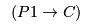and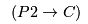need to be false ....
3. Rewrite both statements using the law of contrapositive. Is this clearer?
4. Directly prove that ifis true, thenis true. You might need cases Assume eitheror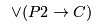is true .....
5. It is difficult to prove the converse statement, if, then. Give it a go! Focus on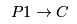, consider the possibilities Eitheris true, or it is false. One case is easy ..

## Quantifiers

1. Explain why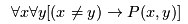allows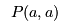to be true.
1. Negate and simplify, what does this say?
2. Suppose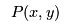is true iff x and y are pals. Write an English statment equivalent to this. Everyone is pals with everyone else.
2. What does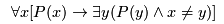say? For every P thing, there is another (different) P thing.
1. What is the negation of the previous statement?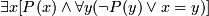2. The predicate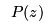can be true or false.
1. For how many z can canbe true? Any number but one. If only one z hadbeing true, there wouldn't be a different y as required.
2. How many can be false? Any number.

## Relations

1. We've discussed four properties that relations can have.
 reflexive symmetric anti-symmetric transitive
1. Give an example of a relation that has all four properties.
The equality relation.
2. Wait! There is a relation that is both an equivalence relation and a partial order? What must the Hasse diagram of such a relation look like?
A graph with no edges.
3. Give an example of a symmetric and transitive relation that isn't reflexive
The empty relation (on any non-empty set) is one example. Another is where both people have met me (some people have, but not everyone).

## Induction

1. A list of induction problems
2. This is a statement of what induction does, the set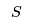is the collection of natural numbers collected by the process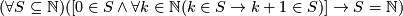This is very complicated!
1. Which part is the base case?
2. Which part is the induction hypothesis and step?
3. The last part is a promise, what is the promise?
4. What is the negation of the statement and what does it say?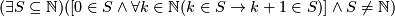There is some subset of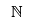for which induction doesn't work, it misses some number.
3. The least number principle (which we haven't seen) says that every non-empty subset ofhas a least element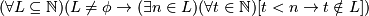1. Which part talks about the set being non-empty?
2. What is the least element?
3. Negate this statement and explain what is says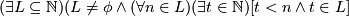There is a subset of, no matter where we think the bottom of the set is, we can always go lower.
4. Use induction to prove that the least number principle is true.
Look at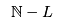, the set of values that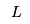doesn't collect
Do induction on, you should find that it satisfies the conditions, so it collects everything ...
4. I claim that, for any fixed natural number C (however large), there is another constant D, for which the following is true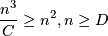1. What is D, in terms of C?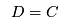will work.
2. Prove the statement is true by induction, using your answer to (a).
• BC Check each side.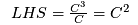and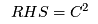. Clearly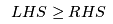• IH. We assume that the statement is true for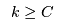i.e.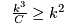• IS. Be prepared to rewrite the induction hypothesis. One of the rewrites will be a good base case Keep dividing through by k.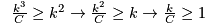Lets do it!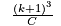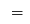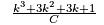Expanding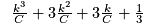Searching for IH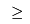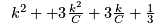By IH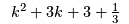Using rewrites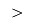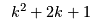Clearly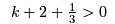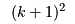Done!

## Graph Theory

1. A forest is a graph with no cycles. A connected graph with no cycles is a tree.
1. Explain (intuitively) why a graph, all of whose edges are bridges, would be a forest? Be contrary, assume there is a cycle. If there were a cycle, then deleting an edge in that cycle would still connect the endpoints, via the rest of the cycle. So no disconnections, hence no new connected components.
2. The converse is trickier, try to explain why a forest consists entirely of bridges Try the contrapostive statement, a graph that isn't entirely made of bridges isn't a forest. Suppose the graph has an edge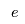which isn't a bridge. So deletingdoesn't disconnect the endpoints. Take the walk from the endpoints (in the graph without) and add on. That is a cycle!
3. A tree is a connected forest, so a forest consists of trees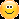Explain why, in a tree,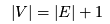.When you delete an edge, one tree becomes two trees The hint implies that recursion (hence induction) should work Supose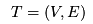denotes the tree in question with vertices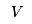and edges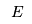. You check the case when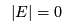. The IH is any tree with less than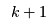edges satisfies the equality. Now suppose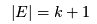, when we delete an edge we get two trees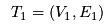andrespectively. Induction step follows

 |V||V_1|+|V_2| Two trees|E_1|+1+|E_2|+1 IH(|E_1|+|E_2|+1)+1 Rearrange so edges come first|E|+1 In brackets are the edges